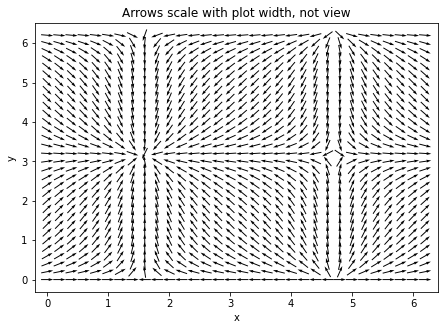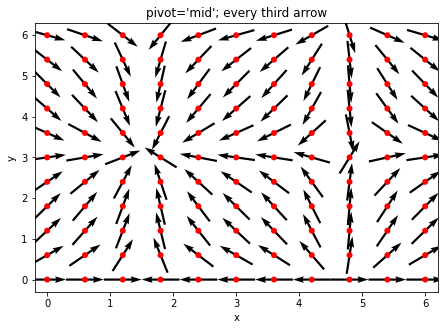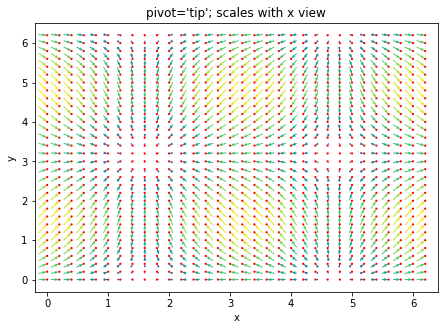# Quiver Demo#

URL: http://matplotlib.org/examples/pylab_examples/quiver_demo.html

Most examples work across multiple plotting backends, this example is also available for:

```import numpy as np
import holoviews as hv
from holoviews import opts

hv.extension('matplotlib')
```## Define data#

```xs, ys = np.arange(0, 2 * np.pi, .2), np.arange(0, 2 * np.pi, .2)
X, Y = np.meshgrid(xs, ys)
U = np.cos(X)
V = np.sin(Y)

# Convert to magnitude and angle
mag = np.sqrt(U**2 + V**2)
angle = (np.pi/2.) - np.arctan2(U/mag, V/mag)
```

## Matplotlib#

```label = 'Arrows scale with plot width, not view'

opts.defaults(opts.VectorField(aspect=1.5, fig_size=200))

vectorfield = hv.VectorField((xs, ys, angle, mag))
vectorfield.relabel(label)
``````label = "pivot='mid'; every third arrow"

vf_mid = hv.VectorField((xs[::3], ys[::3], angle[::3, ::3], mag[::3, ::3]))
points = hv.Points((X[::3, ::3].flat, Y[::3, ::3].flat))

opts.defaults(opts.Points(color='red'))

(vf_mid * points).relabel("pivot='mid'; every third arrow")
``````label = "pivot='tip'; scales with x view"
vf_tip = hv.VectorField((xs, ys, angle, mag))
points = hv.Points((X.flat, Y.flat))

(vf_tip * points).opts(
opts.Points(s=5),
opts.VectorField(color='Magnitude', pivot='tip', magnitude='Magnitude', title=label))
```This web page was generated from a Jupyter notebook and not all interactivity will work on this website. Right click to download and run locally for full Python-backed interactivity.